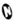Expressions Expressions are an advanced LightWave 3D feature that uses mathematical formulas to modify the value of any animation channel. Expressions let you make the motion of scene items dependent on other item motions or factors in a scene. You could, for example, force an object to stay between two other objects, keep feet from going through the floor, or even control the entire posture of a character based on its feet! The possibilities are endless. There are two types of expression, "Bracket" expressions and “LScript” expressions. Each has their advantages and disadvantages. Bracket expressions take their name from the fact that the channel they are reading is always enclosed in square brackets, “[“ and “]”. The LScript expressions have a syntax similar to that of LScript. You can use much of the LScript documentation to help with the use of the functions available for using in LScript expressions. Note: If you would  a reference of available functions - you can get them from the LScript documentation or from Expression Builder. Bracket Expressions – syntax Bracket expressions always have the channel reference enclosed in square brackets and have the general syntax as shown in the figure.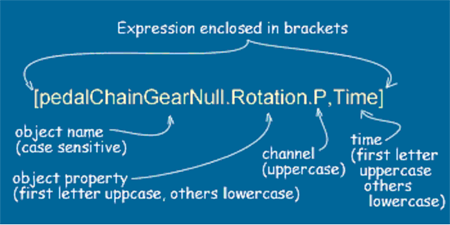You can also use “Frame” in place of the “Time and you can also do things like refer to earlier times e.g. [objectName.Position.X, Time – 0.1] The primary disadvantage of Bracket expressions is that they may only be used via the “Expressions'' tab in the graph editor.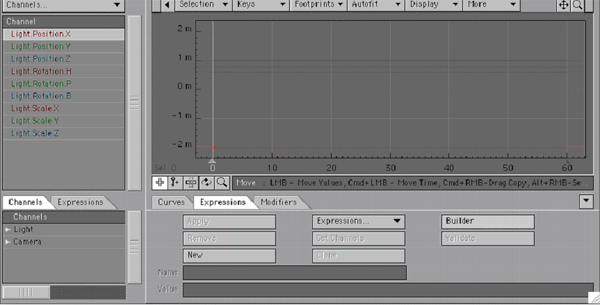They also cannot be used as part any motion that uses an IK calculation. LScript Expressions - syntax LScript expression are not enclosed by square brackets. The object property is all lowercase, as is the channel – see the figure below.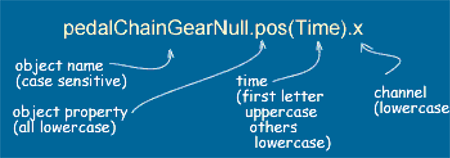LScript Expressions are generally used via the Modifiers'' tab in the graph editor,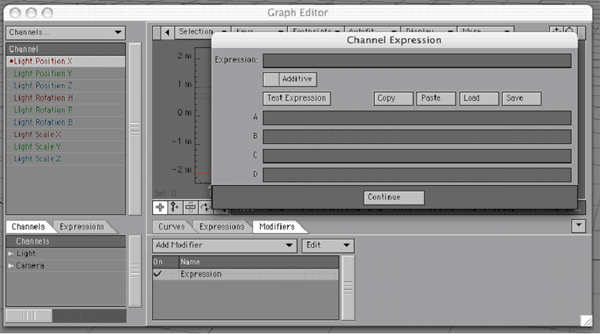or, more typically, via the Motions Options panel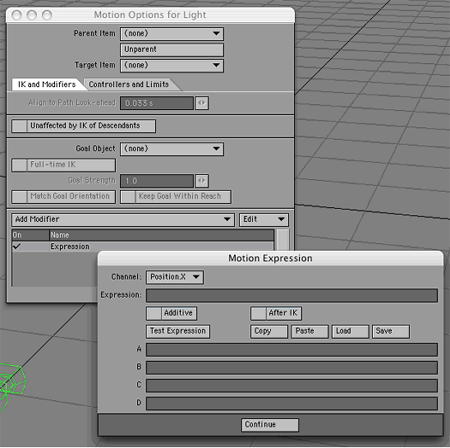One advantage of LScript expressions applied in the Motion Expressions panel is that they can act After an IK example. LScript expressions can also be used to create expression-controlled displacements via the Displacements panel where the expression can act before or after a bone deformation.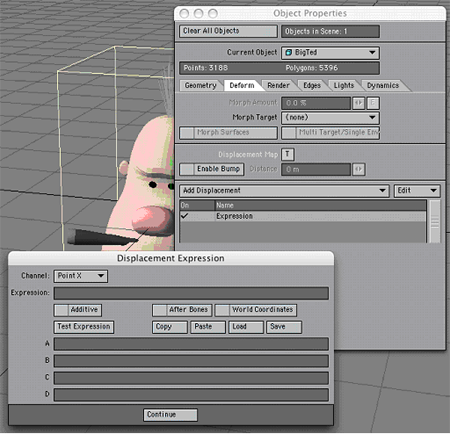Using Expressions – Examples Expression Basics:  Rotating a Gear I've been using LightWave 3D® for many years now and Expressions have always been something that I considered too technical, something that I'd give my math friends to work on. It wasn't until recently that I found out that it's quite simple to use expressions  for many things. This tutorial will walk you thru setting up a basic expression that will help speed up animating gears on a Mech. I promise this wont hurt. :)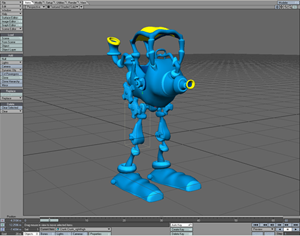Creating an Expression: Step 1: Load the Crunk Car from the Content CD. Our goal in this setup is to have the  gears automatically rotate when the Thigh "Crunk_rightthigh" is rotated. The lower gear "Crunk_Gear01" is already setup to rotate by being parented to the thigh. We can do this because the lower gears pivot point is in the same place as the Thigh. The upper gear "Crunk_Gear02" doesn't share the same pivot point and needs to rotate in the opposite direction. You could manually animate the upper gear but using an expression will save you the hassle. Step 2: Select "Crunk_rightthigh" as the Current Object.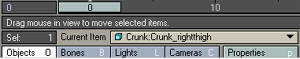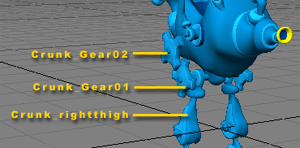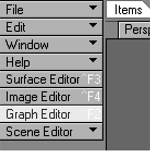Step 3: Open the Graph Editor by clicking on the Graph Editor button. (CTRL +F2)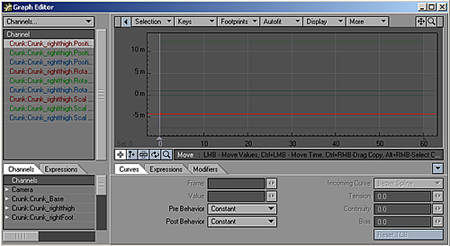Step 4: Click on the Expressions menu tab located under the graph display.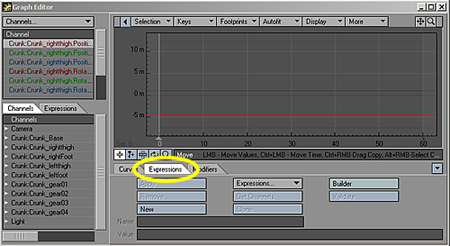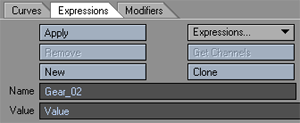Step 5: Click the button labeled "New" and give it a name. I chose to name mine "Gear_02".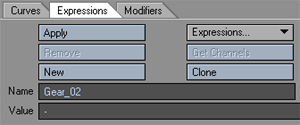Step 6: In the Value field, select "Value" and replace it with "-". The "-" will make the gear spin the opposite way of the thigh by giving it a negative value.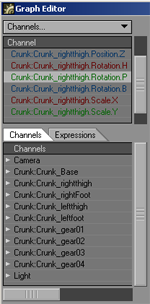Step 7: We want the Gear to spin the opposite way of the Thigh by giving it a negative value of the Thigh's Pitch. The "-" makes it negative, now all we need is the Pitch value of the Thigh. From the Channel List Choose "Crunk:Crunk_rightthigh.Rotation.P".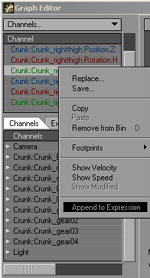Step 8: Right-Click and Choose Append to Expression. The Value Field should look like this: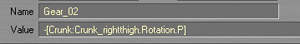Congratulations! You have just written your first expression. Now that wasn't so hard was it? All we need to do now is apply this expression to "Crunk_Gear02" and we'll be finished with the setup. Let's continue.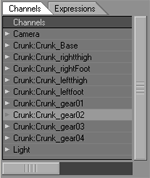Applying an Expression: Step 9: Now that we have our Expression let's apply it to the Gear. From the Channels list select "Crunk_Gear02".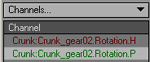Step 10: From the Channel List Choose "Crunk_gear02.Rotation.P". Step 11: Click Apply.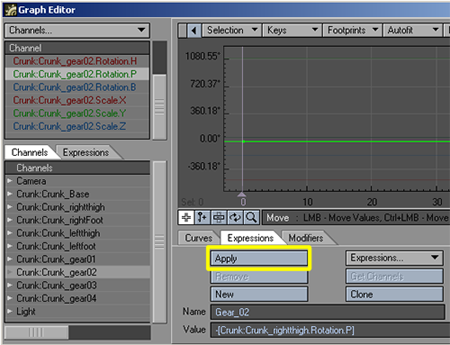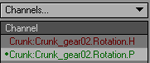Note: A small dot is placed next to the channel to let you know that it has an expression attached to it. Let's take a look at what happens when we rotate the Thigh. Step 12: Close the Graph Editor window and make sure that Auto Key Create is selected.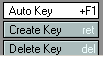Step 13: Select "Crunk_rightthigh" as the Current Object, and rotate it's Pitch.Crunk_Gear02 should rotate on it's Pitch in the Opposite direction of  Crunk_Gear01 and Crunk_rightthigh.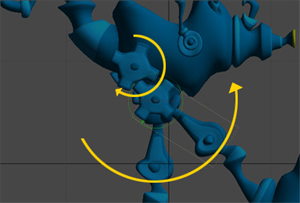Well, there you have it. Your first expression applied and in action. I told you this was going to be easy. We've only scratched the surface on the power of Expressions in LightWave. Expression Builder In this example we will use the Expression builder to center the hips of a character between its two feet. This example assumes the characters hips are not determined by any form of IK calculation. If that is the case you will need to use LScript expressions (applied via the Motion Options panel) as only Lscript expressions can after after IK. Right – lets get to it! Assume the name of the characters two feet are “footLeft” and “footRight” and that the position of the hips are controlled by a single null called “hip”.  To center the hip null we will fix its X and Z coordinates (and leave the height, Y, to the animator). Open the Graph Editor and load the hip channels. Click on the Expressions tab – you panel should look like the figure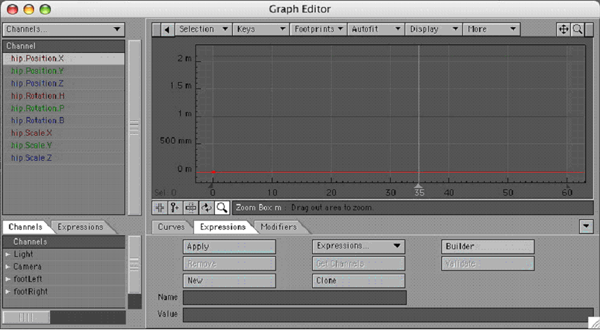If you select your hip null before opening the Graph Editor then the hip channels will automatically be added to the channel bin. First we will create the bracket expression to center the X coordinate of the hip null. Click on the “Builder” button to open the Expression Builder panel, click on the expression template button at the top of the panel and select the "Hip Center (Type A)" menu option as shown below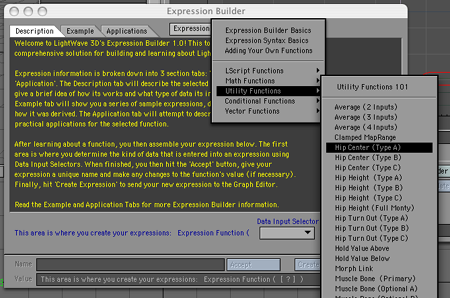This brings up the expression template – all you have to do is say which channels you want to slot in to the template. Expression builder will then create the full expression for you and add it to the Graph Editor. The template for hip centering looks like can be seen below.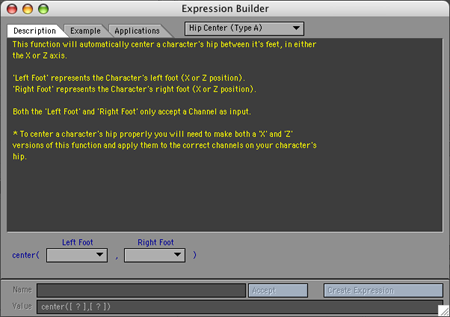The good thing about the Expression Builder (apart from creating a template for you) is that it also gives an explanation for how to use the expression template.  To center the hips we will use the “center” function. This function takes two arguments, the two coordinates between which you want to center the object. The important thing to understand is that the expression can only center in one dimension. To center in two dimensions (ie. the X and Z coordinates) we use the expression twice. Once to center the X coordinate and a second time, using the Z coordinates, to center in the Z direction. Centering in both the Z and X directions at the same time gives you centering in two dimensions, in this case in the XZ plane. Back to the EB template First for the X direction. We need to enter the X position of the left foot and the right foot. To do this for the left foot click the “Left Foot” pop up menu button and select the “channel” option to bring up the channel selection panel as shown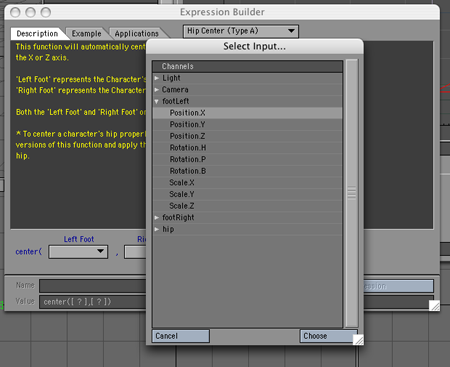and then select the “Position.X” of the footLeft object.  Click “Choose” and you should notice the X position channel of the footLeft object has been entered in to the expression (which is written in the “Value” field on the panel) as shown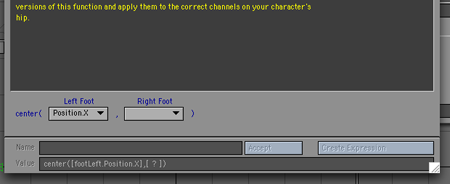Do the same for the “Right Foot” pop up menu, but now select the  Position.X channel for the rightFoot object. The EB panel should now look like this: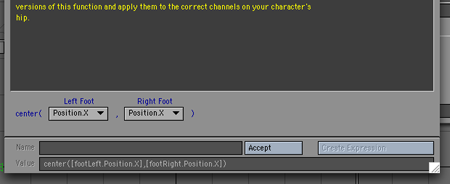Now, click the “Accept” button to accept the expression. EB gives the expression a name in the “Name” field – usually something like “newExp_2” – delete this and replace it with something meaningful like “centerHips_X”. Finally, click “Create Expression” – this tells EB to enter the expression in the expression fields in the Graph Editor. Close EB and the Graph Editor should now contain your expression as shown below.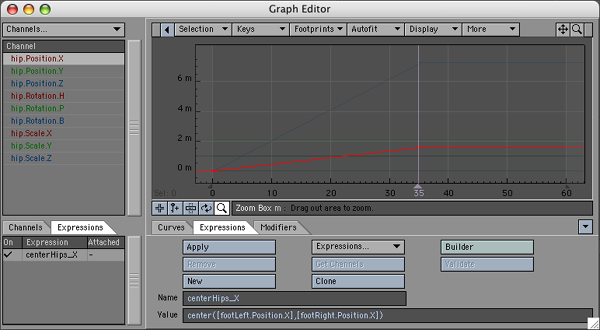In order for the expression to actually affect the X coordinate of the hip object we need to “Apply” the expression the  X channel of the hip object. To do this select the “hip.Position.X” channel in the channel bin and then click the “Apply” button. The hip.Position.X channel should then have a dot to the left of the name to show that that channel has an expression applied to it. If you have the “Always Show Modified” switched on in the Graph Editor options then as soon as you apply the channel the modified curve will appear in the curve window as shown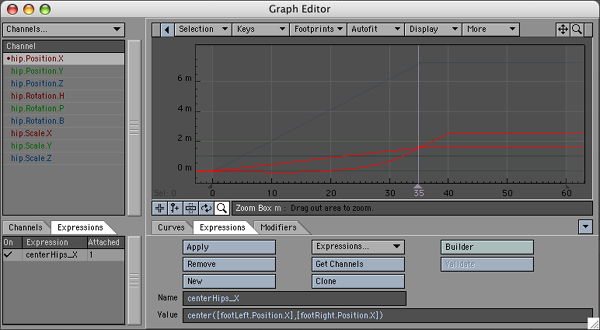We still have to center the Z coordinate of the hip object. To do this you could use the Expression builder again (choosing the Positon.Z channels for the foorLeft and footRight template slots), however, there is a quicker method. Since the expression for the Z coordinate is similar to the X coordinate we can create it by “Cloning” the centerHip_X expression. You do this by clicking the “Clone” button (on the Expression Tab of the Graph Editor). This will create the same expression, but with the new name “centerHip_X (Clone)”. All you need do it edit the expression name – change it to something like “centerHip_Z” and then change the X’s in the actual expression to Z’s. Then select the hip.Position.Z channel in the channel bin and click “Apply” to get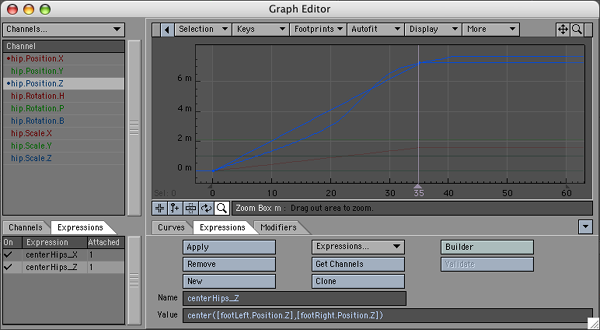That’s it! Your hips will now remain centred between the character feet no what the feet do. If you look at the Utility functions in Expression builder you will notice there are several variations of hip centering which are a little more complex to set up but they give you more control over your character. Joystick Control for morphs Expression Example In this more advanced example we will use Expression Builder to create the expressions for a joystick control. The joystick will be used to control the blinking of a characters eyes. Overview: We begin with a bit of an explanation of the ideas behind what we are trying to do. The blinking of characters eyes is modelled using four morphs: A left eye blink, a right eye blink, both eyes blinking and both eyes opening wide. We will control the left and right eye blinking by moving a null to the left and right respectively. The opening and closong of both eyes will be controlled by the same null, not with the left/right motion, but with the up/down motion of the null. We will call this null the “joystick” null. The big advantage of using this method is that if we move the null both sideways and up (or down) ie diagonally, then we simultaneously combine all the four morphs  by using only one controller – the joystick null. How do we achieve this with expressions? We will use four expressions: blinkLeft, blinkRight, blinkBoth and wideOpen. Each expression will connect the joystick null to one of the four morph channels: object.Body.Eyes.Blink_Left, object.Body.Eyes.Blink_Right, object.Body.Eyes.Blink_Both and object.Body.Eyes.Wide_Both. (The actual object in the screen dumps is called William_Rig.) There remains one further hurdle, when the joystick null moves, it will move a certain distance (eg 50mm), we will need to get the expression to convert this distance in to a morph percentage. When using morphs 100% is actually represented by the number 1 (and 0% by 0). Thus if we want a distance change of 50mm to correspond to a morph percentage change of 0% to 100% we will need to use a bit a maths to convert this range an range of 0 to 1. Actually we won’t need any math phew!) since there is a function  called “maprange” which will do it for us. Now, the joystick it just a null which we will move in the XY plane to control the morphs. Now, the utility of the joystick approach is greatly enhanced if there is some graphical on screen indication of which directions correspond to which morph changes, and also, were 0% position is. For this example we have created all of these using five other nulls. Four nulls are used with an “Item Shape” custom object whose only purpose is to place some text in the viewport. The last null has a square as the custom shape showing the range for the joystick (and the center). Also, all the nulls are parented to the “square window” null. This allows us to position the whole joystick control setup anywhere in the scene. For convenience, the joystick is usually placed in a viewport by itself (or alongside any other such controls)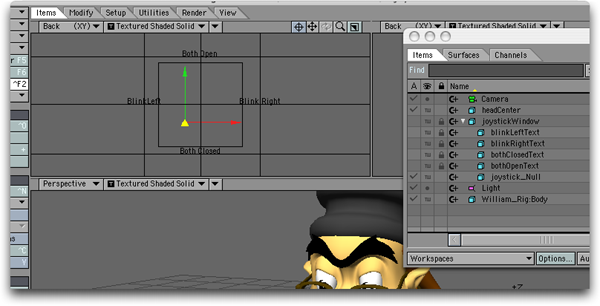Let's get the expressions going. We will start by creating the BlinkLeft expression. This expression will control the closing of the left eye as the joystick moves from O to A (see the figure).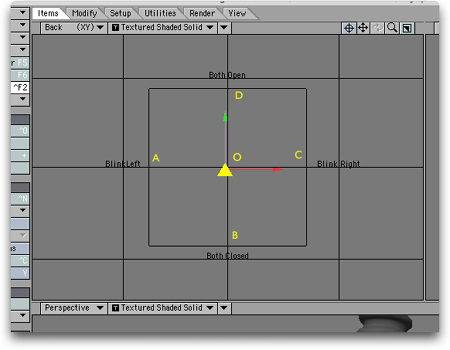In terms of distance the null is moving from X=0 (at O) to X=-50mm (at A) the full range of the eye closing corresponds to a morph percentage change of 0 to 75% or, for the expression, a change from 0 to 0.75.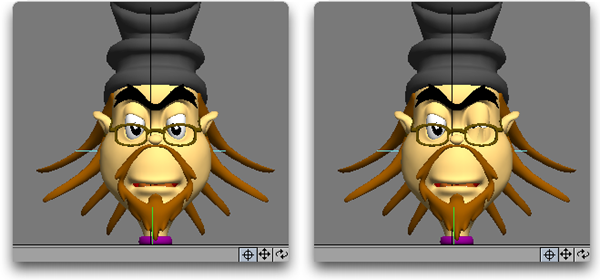We will use Expression Builder (EB), so open the Graph Editor, click the Expression Tab and click the “Builder” button. Once the EB is open use the pop-up menu to choose the “Clamped Range” utility function as shown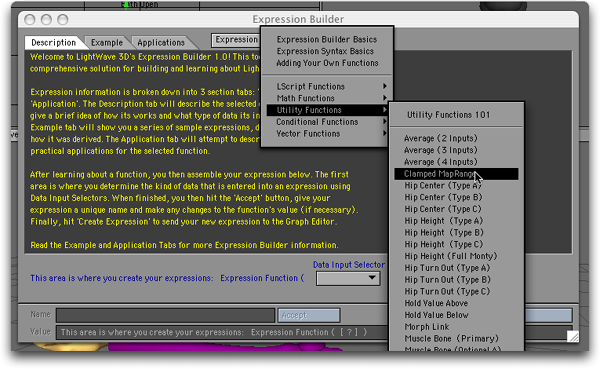We now have an expression template with five inputs.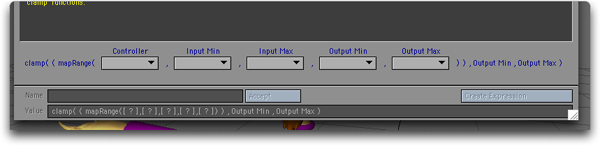This is the function that “maps” the range of the joystick controller (ie. –50mm to 0mm) to the range of the morph (ie 0.75 to 0.0) To use it we need the joystick controller to go in the first input, so, select the “Channel” from the Controller input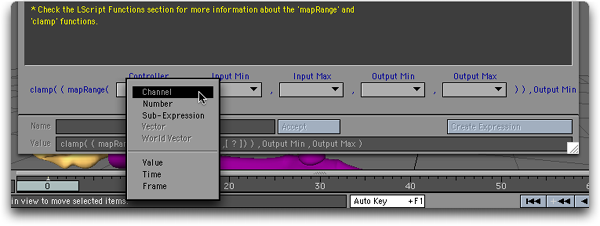and then select the X position channel of the joystickControl object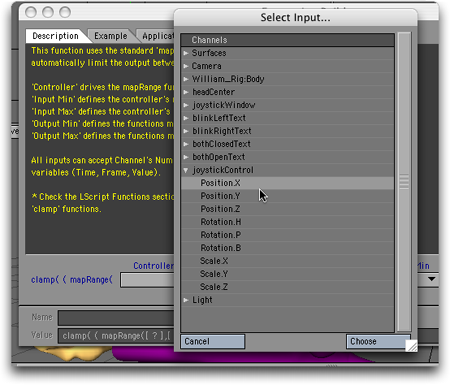Now, the “Input Min” is the minimum value (as a number) that the joystick controller will take. In this case it is –50mm, or –0,05. Thus, choose the number option in the “Input Min” popup,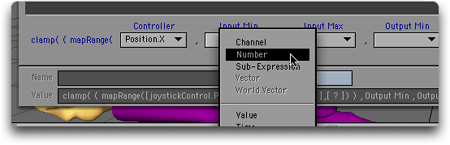and enter –0.05. Similarly, for the remaining three entries: The “Input Max” is the maximum value we expect the X coordinate of the joystick to take (for controlling the left blink – it will actually move to +50mm for the right blink, but for the left blink we want an X value of 0 to correspond the 0 morph percent. Thus the “Input Max” is 0. For the “Output Min” we want 0.75 – the morph percent that corresponds to –50mm (which isn’t actually the smallest number, and for the “Output Max” we want 0 (giving a morph percent of 0%). Once all your number are entered the template should look like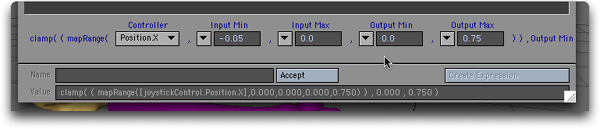Now, click the “Accept” button, which tells EB to accept all your template entries. EB usually gives the expression some generic name; so delete it and type in “blinkLeft” for the expression name.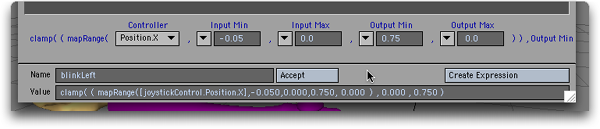Finally, click the “Create Expression” button. This tells EB to send the expression to Lightwave. If you now open the Graph Editor and click on the Expression Tab you should see your expression!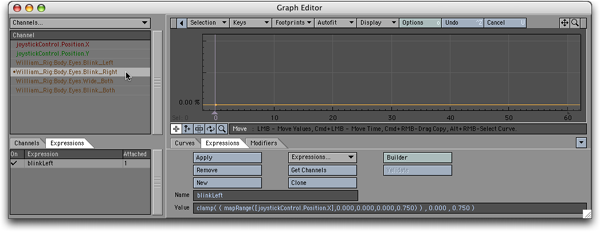You can close the EB panel as we won’t need it till later. Back to the Graph Editor (GE). We now have to attach or apply the expression to the channel which it is going to control ie the left blink morph channel. To do this you need to get the channel in to the channel bin (the list on the top left of the GE).  In our scene the morph channel is called William_Rig:Body.Eyes.Blink_Left”. Once it is in the channel bin select it and then click the “Apply” button on the Expression Tab. A dot should appear next to the morph channels name telling you it now has an expression attached to it.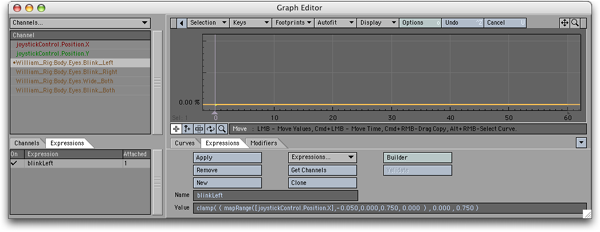That’s it for the left eye blinking. If you move the joystick controllers X position from –50mm to 0 the left eye should blink. We now have to repeat three more times to create the expression to control the right blink, the wide open case and the both closed case. The process is exactly the same with only the numbers entered in to the Expression builder changing and, for the blinkboth and wideOpen cases, it is the Y position of the controller that is used. These three EB panels are show below Blink right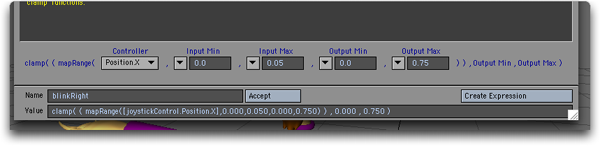Blink both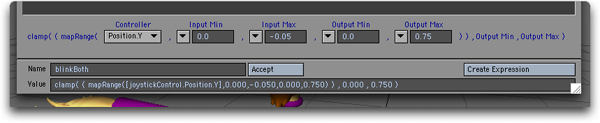Wide Open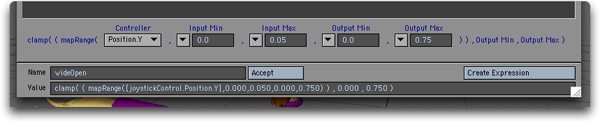Once you have applied these three expressions to their respective channels your joystick controller should be fully functional. One last thing. Why is the “clamp” function part of each expression? Well, the map range function can take values outside the range of the “output” min and max values i.e. can give negative morph percents or percents greater than 100%. This is what the clamp function stops happening. It takes in the values of the map range function as forces then to stay in the range of the very last two numbers in each expression (in this case, the range 0 to 0.75) Connecting LScripts to Expressions You can write an Lscript function, or user defined function (UDF), using all the keywords available in LScript and then access the function in an expression. Employing Embedded LScript, users can now write their own functions for use with LightWave Expressions. Expressions UDFs are used just like any built-in Expressions function.  Parameter passing is limited to simple data types--strings, numbers and vectors.  As long as an expression evaluates to one of these data types, it can be used as an argument. Expressions UDFs are stored in the LighjtWave->LScripts directory within their own directory called "expressions".  A default library of functions can be maintained within this directory called "library.ls".  This library of functions is automatically loaded into the Expressions engine when LightWave is initialized, and its defined functions are consequently available to any LightWave expression or Expressions UDFs that references them. Additionally, individual Expressions UDFs can be stored in their own files in this same directory. The name of the file containing the UDF must exactly match that of the function name being referenced.  The file may contain any number of other UDFs to support the main function, but must contain at least a UDF whose name and argument count matches that being referenced in the expression. Data exchange between UDFs is not limited in their types.  UDF-to-UDF calling is exactly the same as it is in LScript. By way of example, assume the following files exist in the required directory:          LightWave->LScripts->expressions->library.ls         LightWave->LScripts->expressions->channelValue.ls   The "library.ls" file contains the following content:         locateChannel: fullchannel        \{             parts = parse(".",fullchannel);             group = ChannelGroup(parts);  // start with root channel group             lastgroup = group;             subgroup = nil;             x = 2;             while(group)            \{                 // scan sub-groups to match parts[x]                 // if a match can't be found, then it                 // is probably the start of the channel                 // name                 subgroup = ChannelGroup(group,subgroup);                 last if !subgroup;                 if(subgroup.name == parts[x])                 \{                     group = subgroup;                     lastgroup = group;                     subgroup = nil;                     ++x;                 \}             \}             if(!lastgroup) return(nil);             // anything left in the parts[] array are the components             // of the channel name itself.  put them together for             // channel searching             channelname = "";   // avoid creating an array             psize = parts.size();             while(x <= psize)             \{                 channelname += parts[x];                 if(x < psize) channelname += ".";                 ++x;            \}             // scan the defined channels in the final group to see             // if we can match the channel name             chchannel = lastgroup.firstChannel();             while(chchannel)             \{                 last if chchannel.name == channelname;                 chchannel = lastgroup.nextChannel();             \}             return(chchannel);         \}         // replace the built-in clamp() function         clamp: val, lower, upper        \ {                                  result = val;             if(val < lower)      result = lower;             else if(val > upper) result = upper;             return(result);         \}   While the "channelValue.ls" file contains the following content:         chan;         chanName;         channelValue: channel, time         \{             if(chanName != channel) chan = nil;             if(!chan)             \{                 // cache the channel for speed                 chan = locateChannel(channel);                 if(!chan) return(0);                 chanName = channel;             \}             return(chan.value(time));         \}   In Layout, you might then enter an expression like:         clamp(channelValue("WashLight.Intensity",Time),0.0,1.0) This will invoke the channelValue() UDF, which then invokes the locateChannel() UDF (defined in the default library file "library.ls") to resolve a string channel reference to an actual LScript Channel Object Agent.  The channelValue() UDF returns the value of the  specified Light Object's intensity value at the current time.  This value is then passed to  the (script) clamp() UDF (also defined in "library.ls") to keep it in a specified range. Alternately, you could use the Graph Editor's direct channel reference syntax with the UDF call:         clamp([WashLight.Intensity,Time],0.0,1.0) UDF references that have been loaded into the Expressions engine are automatically updated the next time they are evaluated when their respective files have been modified.  For instance, if you have expressions referencing channelValue(), altering the last line of the function to   read:             return(chan.value(time) + 1.0);   will instantly return new values the next time the expression is evaluated (e.g., on the next frame).  \end{document}  Object References Objects are referenced by their name. The system does not currently suport space characters (' ') in object names, so cloned objects (i.e., "Null (1)", "Null (2)", etc.) cannot be used unless they are renamed. The "Scene" object is the only pre-defined object in the system. All other object references must equate to an object in the current scene. Built-in Functions double                                  sqrt(double) double                                  exp(double) double                                  log(double) double                                  sin(double) double                                  cos(double) double                                  tan(double) double                                  asin(double) double                                  acos(double) double                                  atan(double) int                                        random(int,int) double                                  vmag(vector | double,double,double) (int | double | vector)              abs(int | double | vector) double                                  ceil(double | vector) double                                  floor(double) double                                  cosh(double) double                                  sinh(double) double                                  tanh(double) double                                  mod(double | vector,double | vector) double                                  pow(double,double) double                                  rad(double | vector) double                                  deg(double | vector) (double | int | vector)              max(double | int | vector,double | int | vector) (double | int | vector)              min(double | int | vector,double | int | vector) double range                        (double,double,double) double                                 selector(double,double,double,double) double                                 step(double,double,double) double                                 round(double,int) double                                 frac(double) double                                 fac(double) double                                 cot(double) double                                 sec(double) double                                 csc(double) vector                                  cross3d(vector,vector) double                                 dot3d(vector,vector) double                                 cross2d(double,double,double,double) double                                 dot2d(double,double,double,double) vector                                  normalize(vector) vector                                  center(vector,vector) vector                                  extent(vector,vector) string                                   parse(string,string) double                                 number(string) vector                                  vector(string) string                                   string(double + int + string + vector) int                                       integer(double | string) string                                  strleft(string,int) string                                  strright(string,int) string                                  strsub(string,int,int) string                                  strupper(string) string                                  strlower(string) double                                 randu([double | int]) string                                  hex(int[,int[,int | "true" ]]) string                                  octal(int[,int[,int | "true" ]]) double                                angle(vector,vector,int) Common Object Methods and Data (all objects respond) vector                                    pos(double) vector                                    position(double) vector                                    rot(double) vector                                    rotation(double) vector                                    right(double) vector                                    up(double) vector                                    forward(double) vector                                    pivot(double) vector                                    wpos(double) vector                                    wposition(double) vector                                    wright(double) vector                                    wup(double) vector                                    wforward(double) vector                                    limits.pos.min vector                                    limits.pos.max vector                                    limits.position.min vector                                    limits.position.max vector                                    limits.rot.min vector                                    limits.rot.max vector                                    limits.rotation.min vector                                    limits.rotation.max vector                                    limits.right.min vector                                    limits.right.max vector                                    limits.up.min vector                                    limits.up.max vector                                    limits.forward.min vector                                    limits.forward.max vector                                    limits.pivot.min vector                                    limits.pivot.max vector                                    limits.wpos.min vector                                    limits.wpos.max vector                                    limits.wposition.min vector                                    limits.wposition.max vector                                    limits.wright.min vector                                    limits.wright.max vector                                    limits.wup.min vector                                    limits.wup.max vector                                    limits.wforward.min vector                                    limits.wforward.max Mesh Object Methods and Data double                              dissolve(double) int                                    points int                                    polygons Light Object Methods and Data vector                                    color(double) int                                          points int                                          polygons double                                    coneangle.rad double                                    coneangle.radius double                                    coneangle.edge Camera Object Methods and Data double                                    zoom(double) double                                    zoomfactor(double) double                                    focallength(double) double                                    focaldistance(double) double                                    fstop(double) double                                    blurlength(double) double                                    fovhor(double) double                                    fovhorizontal(double) double                                    fovver(double) double                                    fovvertical(double) Scene Object Methods and Data int                                    points int                                    polygons int                                    renderstart int                                    renderend int                                    renderstep double                              fps int                                    width int                                    renderwidth int                                    height int                                    renderheight double                              aspect double                              pixelaspect double                              aspectratio int                                    minspp int                                    maxspp int                                    recursion int                                    maxrecurse int                                    recursedepth int                                    usingTraceShadows int                                    usingTraceReflection int                                    usingTraceRefraction int                                    usingFields int                                    usingEvenFields int                                    usingMotionBlur int                                    usingDOF int                                    usingLR int                                    usingLimitedRegion int                                    lr.x1 int                                    lr.left int                                    lr.x2 int                                    lr.right int                                    lr.y1 int                                    lr.top int                                    lr.y2 int                                    lr.bottom int                                    limitedregion.x1 int                                    limitedregion.left int                                    limitedregion.x2 int                                    limitedregion.right int                                    limitedregion.y1 int                                    limitedregion.top int                                    limitedregion.y2 int                                    limitedregion.bottom Selector/Converter items double                                    x (selects the first element of a multiple-data type) double                                    y (selects the second element of a multiple-data type) double                                    z (selects the third element of a multiple-data type) int                                           r (selects the first element of a multiple-data type) int                                           g (selects the first element of a multiple-data type) int                                           b (selects the first element of a multiple-data type) vector                                     rbg (converts a vector data into color-normalized data) string                                     asStr (converts int, double, vector to string) string                                     asString int                                         asInt (converts string, double to integer) int                                         asInteger double                                    asNum (converts string, int to double) double                                    asNumber vector                                     asVec (converts int, double, string to vector) vector                                     asVector Note: A vector is a group of related values. They could relate to position (x,y,z), rotation (h,p,b), color (r,g,b), etc. To get only one component, use a selector as demonstrated below. Note: Expressions react to interactively moved items, even if Auto Key is turned off. Note: You may use XS, YS, and ZS as aliases for Scale.X, Scale.Y, and Scale.Z. Sample Expressions HeadLight.rot(Time).h returns the heading rotation value of the HeadLight item at the current time. Left.pos(Time).x + Right.pos(Time).x returns the sum of the Left and Right items positions on the x axis. <1,2,3>.y returns 2 <1,1,1>.rgb returns <255,255,255> <.5,.25,1>.rgb returns <127,63,255> BackLight.color(frame / Scene.fps).rgb returns RGB vector value for color BackLight at a user-defined frame converted to a time index using the Scene object's fps setting. The frame variable is returned to the caller and can have its value explicitly set before each evaluation of the expression. 2 * "1 2 3".asVec.y returns 4 ((Scene.usingLR ? (Scene.lr.right - Scene.lr.left) : Scene.width) / 2).asInt finds the horizontal center of the frame.